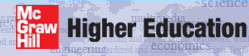# Institut für Thermodynamik der Luft- und Raumfahrt - Universität StuttgartMechanical Engineering - The University of Texas at Austin# integration control

The primary controls for integration of the transport equations include being able to define the physical flow domain for integration and the integration stepsize. The entrainment concept does not exist for internal flows because the flow domain is fixed and there is no possibility of dynamic enlargement or contraction of the domain.

Please read the section overview: numerical accuracy to further understand how the user can affect the solution with respect to stepsize and grid.

Physical Flow Domain - For internal flows, the physical cross-flow domain extends from the surface to the centerline for pipes and thermally-symmetrical parallel-planes channels (kgeom=4,5) or from surface to surface for thermally-asymmetrical parallel-planes channels and annuli (kgeom=6,7). The axial extent of the flow domain is defined for TEXSTAN using the boundary condition array x(m) and there will be nxbc values within the array, where m = 1, nxbc. For example, flow in a pipe will have a domain that typically extends from the pipe flow entry point, and nxbc=2 could be used as a minimum if u is constant over the surface and the surface is either isothermal (constant temperature) or constant heat flux. If there is a need to specify a surface temperature distribution (or surface heat flux distribution) or u = u(x), then more boundary condition locations will be required.

Integration Start and Stop Locations - These are defined by the xstart and xend variables. The starting location of the integration is xstart and the ending location of the integration is xend. The only restrictions are that they be bound within the boundary condition array x(m).

Integration Stepsize - In TEXSTAN the stepsize is computed as a percentage of the shear layer thickness. For external flows the shear layer thickness is the classical boundary layer thickness, and for internal flows it depends on the geometry. For pipe flows the shear layer thickness is the pipe radius, and for the parallel planes channel it is the channel half-width. For the annulus it is the average of the distance between the inner and outer surfaces. The stepsize is then computed as a percentage of however the shear layer thickness is defined.

The input variable that sets the percentage stepsize is deltax. Thus a value =0.1 implies a forward integration stepsize of 10 percent of the shear layer thickness. Such a small stepsize is required because the numerical scheme for TEXSTAN is semi-implicit, rather than fully implicit. The only time this variable can be made larger is in the fully-developed flow region of pipes and channels.

Internal flows such as the combined entry flow in pipes and channels begin with flat profiles for velocity and temperature, so the developing shear layer (boundary layer) is a really a very small percentage of this grid in the beginning. If deltax is set small enough to resolve these extremely high wall gradients in the beginning part of the entry region, the total number of integration steps will be massively large by the time the entry flow is complete and fully-developed flow begins. To overcome this issue for internal flows, the user can set deltax =0.0 and set the control flag kdx =1. With this flag set, values for deltax are now contained in the aux1(m) variable array. This array is then linearly interpolated as a function of x, and the resulting value has precisely the same meaning as deltax.

Note that there is another flow condition which requires variation in stepsize: regions of fast-changing thermal boundary conditions. For example, if the wall heat flux over the first 50% of a pipe flow problem is adiabatic, and the last 50% is a prescribed heat flux for heating or cooling, then numerical accuracy for resolving the fast rate of change of the surface temperature immediately after the step-change in the surface heat flux would require a very small stepsize close to the step and the stepsize could be increased further downstream.

For laminar internal flows, the hydrodynamic entry region correlates logarithmically with (x/Dh)/ReDh , so this becomes an easy way of estimating how to create a distribution of x-locations for varying aux1. For example, one could choose the set of points (x/Dh)/ReDh = 0.0005, 0.005, 0.025 and start aux1(m) at a very low value (say =0.01) and in the region 0-0.0005-0.005 increase the nondimensional stepsize aux1 to 0.25 and then further increase it to a larger value (say 1.0) in the region 0.005-0.025, and then it can remain constant for larger x. The hydrodynamically fully-developed value for (x/Dh)/ReDh is about 0.1. For heat transfer it is similar, but the definition is modified to be (x/Dh)/(ReDhPr).

(x/Dh)/ReDh  aux1
0.0  0.01
0.0005  0.01
0.005  0.25
0.050  1.00

For turbulent internal flows, the hydrodynamic entry length of about 10-40 hydraulic diameters (Dh), so we need a number of integration points within this region. As a rule of thumb, we choose a set of x locations that include x/Dh = 0, 5, 50, 100, and we vary the integration stepsize in this entry region to have a small stepsize as the boundary layers begin. For the region from x/Dh =0 to x/Dh = 5 the stepsize (aux1 value) should be 0.01, and for the interval from x/Dh =5 to x/Dh = 50 the stepsize will linearly increase from 0.01 to 0.1, and for the interval from x/Dh =50 to x/Dh = 100 it will further increase to 0.20, and then remain constant beyond this value. This rule is especially important for two-equation turbulence models.

(x/Dh)  aux1
0  0.01
5  0.01
50  0.1
100  0.2

website updated Sept 2010   © 1996-2010 Michael E. Crawford - all rights reserved - website validated for CSS 2.1 and XHTML 1.0 strict at www.w3.org